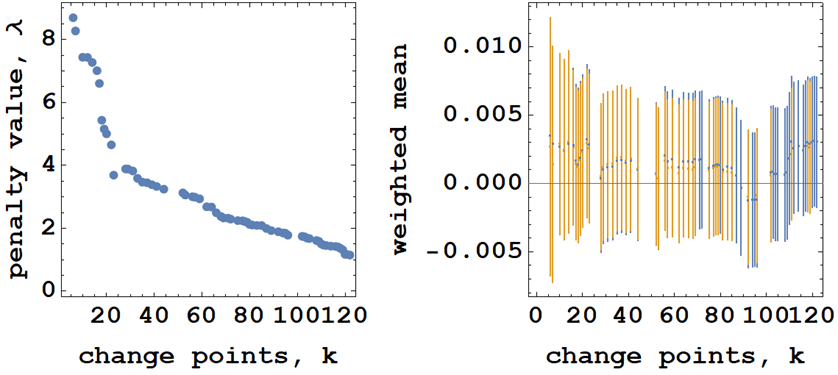# #StackBounty: #mean #weighted-mean What is the best way to combine mean and standard errors calculated from the same data set, but with…

### Bounty: 50

If I have a data set of sample size $$N$$ and I calculate a mean and standard error from this data set using different approaches — for example, if I bin the data into groups by some criteria, and calculate a mean and S.E, I would combine these means and S.E. using a weighted mean approach. If I then change my criteria (which may change the group size for example), I will produced different weighted means.

If I do not have a justification of choosing a particular criterion, an my weighted means are similar, it would seem that the best approach is to combine them into a single statistic. How would I combine these weighted means into a single mean and error?

It would seem to me that a weighted mean of weighted means would be inappropriate, and would give an artificially reduced standard error — especially if I have performed many different analysis strategies — and the same with other approaches of combining studies such as DerSimonian and Laird.

The best Approach I can think of is to take the arithmetic mean of the means and the arithmetic means of the standard errors, as this will not artificially reduce the standard error.

Some context…

I am using change-point analysis to divide some data into groups. I then calculate a mean and S.E for each group and then combine these using a weighted average (I also use REML methods). However, a given weighted mean will be associated to a certain number of change-points, $$k$$, which in turn has an associated penalty value, $$lambda$$.

The question is, which penalty factor/number of change-points is best (or best describes my data). Below we can see a plot of $$lambda(k)$$ and the weighted mean as a function of $$k$$.What I note is after the elbow point feature of the $$lambda(k)$$ is that the weighted mean appears to be quite stable, it therefore seems reasonable to me to combine these weighted means in an appropriate way, given that I have no way to reasonably say "the $$k$$th change-point is the best".

Taking the weighted mean of weighted means is clearly inappropriate as I will be artificially inflating my sample size, hence the S.E. of the weighted mean of weighted means is artificiality reduced.

I have read about various methods of "finding the elbow" in the $$lambda(k)$$ plot, but this seems to be vague and hand-wavy (if I am wrong with that assertion, I am most willing to be educated!)

Get this bounty!!!

This site uses Akismet to reduce spam. Learn how your comment data is processed.